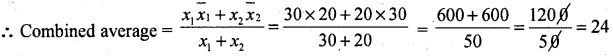# 1st PUC Basic Maths Question Bank Chapter 10 Averages

Students can Download Basic Maths Chapter 10 Averages Questions and Answers, Notes Pdf, 1st PUC Basic Maths Question Bank with Answers helps you to revise the complete Karnataka State Board Syllabus and score more marks in your examinations.

## Karnataka 1st PUC Basic Maths Question Bank Chapter 10 Averages

Question 1.
A student asked to find the arithmetic mean of the numbers 4, 19, 17, 12, 34,16, 7, – 23,18, 21, 25, and x. Find the mean table 18. What should be the number in place of x.18 = $$\frac{196+x}{2}$$ = 216 = 196 + x
∴ x = 216 – 196 = 20

Question 2.
The average of 30 results is 20 and the average of other 20 results is 30. Find the average of results taken to gather.
Given n1 = 30, x̄1 = 20, n2 = 20 , x̄2 = 30Question 3.
If the average of daily wages of workers of two factories is Rs. 53 and average wages to factory ‘A’ with 250 employees is Rs. 50. Find the average wage of factoring ‘B’ with 200 employees.
Given X̄ = 53, X̄A = 50, nA = 250, X̄B = 7, nB = 200i.e., 200 X̄B = 23850 – 12500 = 11,350 ∴ X̄B = $$\frac{11,350}{200}$$ = 56/75
∴ Average wage of factory B = Rs. 56.75Question 4.
Ten years ago the average age of the family of 4 members was 24 years. Two children have been born. The average age of the family is same to day. Find the present age of two children assuming that the children’s age differ by 2 years.